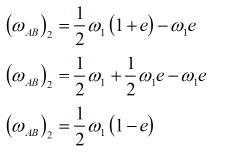# A slender rod CDE of length L and mass m is attached to a pin support at its midpoint D. A second and identical rod AB is rotating about a pin support at A with an angular velocity ω1 when its end B strikes end C of rod CDE. Denoting by e the coefficient of restitution between the rods, determine the angular velocity of each rod immediately alter the impact.

Question-AnswerCategory: Engineering MechanicsA slender rod CDE of length L and mass m is attached to a pin support at its midpoint D. A second and identical rod AB is rotating about a pin support at A with an angular velocity ω1 when its end B strikes end C of rod CDE. Denoting by e the coefficient of restitution between the rods, determine the angular velocity of each rod immediately alter the impact.

A slender rod CDE of length L and mass m is attached to a pin support at its midpoint D. A second and identical rod AB is rotating about a pin support at A with an angular velocity ω1 when its end B strikes end C of rod CDE. Denoting by e the coefficient of restitution between the rods, determine the angular velocity of each rod immediately alter the impact.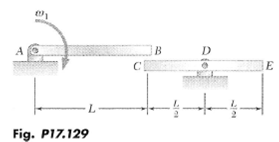Step 1

Given data
Mass of the rod = m
Coefficient of restitution = e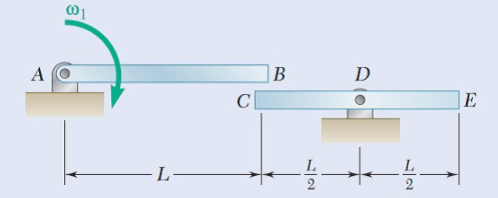To draw the impulseâ€“momentum diagrams that are needed to determine the angular velocity of each rod immediately after the impact.

Step 2

Momenta diagram for the rod ABApply momenta principle before impulse, at the impulse and after the impulse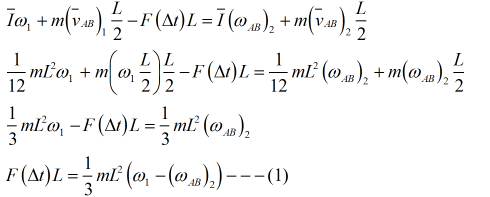Step 3

Momenta diagram for the rod CD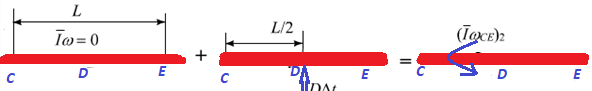Considering the moment at D and substitute impulse equation in (1)Equation for angular velocity of the rod AB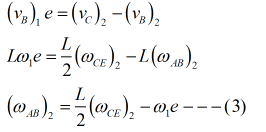Step 4

Calculation of angular velocity of the rod CECalculation of angular velocity of the rod AB